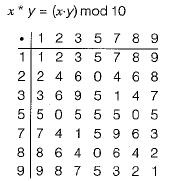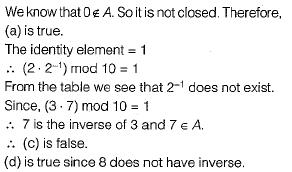Courses

# Set Theory And Algebra (Basic Level) - 1

## 10 Questions MCQ Test Mock Test Series for Electrical Engineering (EE) GATE 2020 | Set Theory And Algebra (Basic Level) - 1

Description
This mock test of Set Theory And Algebra (Basic Level) - 1 for GATE helps you for every GATE entrance exam. This contains 10 Multiple Choice Questions for GATE Set Theory And Algebra (Basic Level) - 1 (mcq) to study with solutions a complete question bank. The solved questions answers in this Set Theory And Algebra (Basic Level) - 1 quiz give you a good mix of easy questions and tough questions. GATE students definitely take this Set Theory And Algebra (Basic Level) - 1 exercise for a better result in the exam. You can find other Set Theory And Algebra (Basic Level) - 1 extra questions, long questions & short questions for GATE on EduRev as well by searching above.
QUESTION: 1

### Let S be an infinite set and S1, S2, ... Sn be sets such that S1 ∪ S2 ∪ .... ∪ Sn = S. Then

Solution: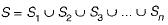For S to be infinite set, atleast one of sets Si must be infinite, since if all Si w ere finite, then S will also be finite.

QUESTION: 2

### Let A be a finite set of size n. The number of elements in the power set of A x A is

Solution:

Number of elements in A x A = n2
∴ Number of elements in the power set of
A x A =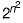QUESTION: 3

### The number of elements in the power set P(S) of the set S = {(φ), 1, (2, 3)} is:

Solution:

if a set has. n elements then its powers set has 2n elements.
Given set S =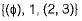Number of elements in S = 3
∴ The number of elements in powerset (S)
= 23
= 8

QUESTION: 4

Suppose X and V are sets and |X| and |Y| are their respective cardinalities. It is given that there are exactly 97 functions from X to Y. From this one can conclude that

Solution:

X and Y are sets.
The card in a lities of X and Y are |X| and |Y|  respectively.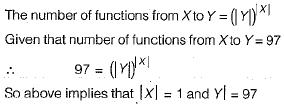QUESTION: 5

Suppose A is a finite set with n elements. The number of elements in the Largest equivalence relation of A is

Solution:

A is a finite set with n elements. The largest equivalence relation of A is the cross-product A x A and the number of elements in the cross product A x A is n2.

QUESTION: 6

The number of functions from an m element set to an n element set is

Solution:

The number of functions from an m element set to an n element set is nm.

QUESTION: 7

The number of binary relations on a set with n elements is

Solution:

The maximum number of elements in a binary relation on a set A with n elements = Number of elements in A x A = n2.
Each element has two choices, either to appear on a binary relation or doesn’t appear on a binary relation.
∴ Number of binary relations =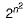QUESTION: 8

The binary relation S = f (empty set) on set A = {1,2, 3} is

Solution:

The empty relation on any set is always transitive and symmetric but not reflexive.

QUESTION: 9

The number of different n x n symmetric matrices with each element being either 0 or 1 is: [Note: power (2, x) is same as 2X]

Solution:

In a symmetric matrix, the lower triangle must be the mirror image of upper triangle using the diagonal as mirror. Diagonal elements may be anything. Therefore, when we are counting symmetric matrices we count how many ways are there to fill the upper triangle and diagonal elements. Since the first row has n elements, second (n - 1) elements, third row ( n - 2) elements and so on upto last row, one element.
Total number of elements in diagonal + upper triangle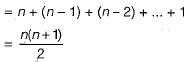Now, each one of these elements can be either 0 or 1. So total number of ways we can fill these elements is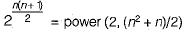Since there is no choice for lower triangleelements the answer is power (2, {n2 + n)/2).

QUESTION: 10

The set {1, 2, 3, 5, 7, 8, 9} under multiplication modulo 10 is not a group. Given below are four possible reasons. Which one of them is false?

Solution:

Let A = { 1, 2, 3, 5, 7, 8, 9}
Construct the table for any x, y ∈ A such that